Homework Help Question & Answers

# Balance the following chemical equation and determine the number of moles of Fe(s) produced when 0.75...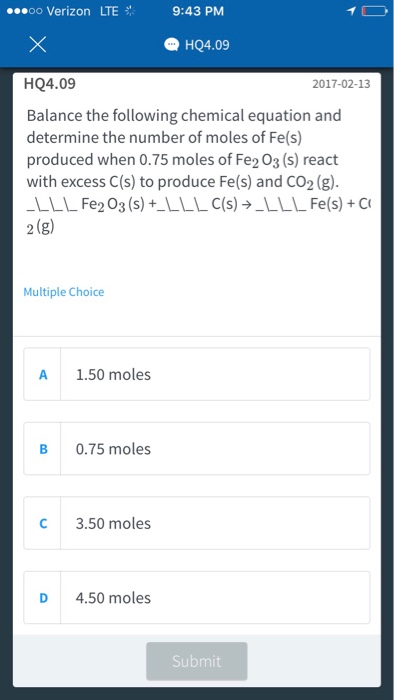Balance the following chemical equation and determine the number of moles of Fe(s) produced when 0.75 moles of Fe_2O_3 (s) react with excess C(s) to produce Fe(s) and CO_2(g). Multiple Choice 1.50 moles 0.75 moles 3.50 moles 4.50 moles Submit

#### Homework Answers

Answer #1

2Fe2O3(s) + 3C(s) --------------> 4Fe(s) + 3CO2 (g)

First balance the number of oxygens multiplying by 3 x CO2 and 2 x Fe2O3 followed by carbon and Fe in the last step.

2Fe2O3(s) + 3C(s) --------------> 4Fe(s) + 3CO2 (g)

Stoichiometrically, 2 mol 3 mol 4 mol 3 mol

2 moles of Fe2O3 react with excess C to produce 4 moles of Fe

1 mole of Fe2O3 reacts with excess C to produce 4/2=2 moles of Fe

0.75 moles of Fe2O3 react with excess C to produce 2*0.75= 1.50 moles of Fe

Answer is A

:-)

Know the answer?
Your Answer:

#### Post as a guest

Your Name:

What's your source?

#### Earn Coin

Coins can be redeemed for fabulous gifts.

Not the answer you're looking for? Ask your own homework help question. Our experts will answer your question WITHIN MINUTES for Free.
Similar Homework Help Questions
• ### Balance the following chemical reaction and determine the number of moles of HI produced when 2.33...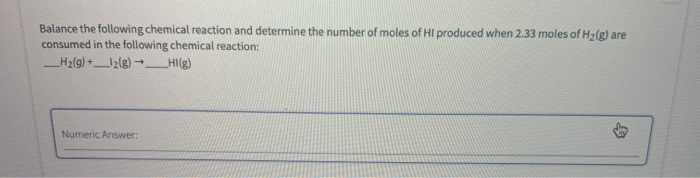Balance the following chemical reaction and determine the number of moles of HI produced when 2.33 moles of H2(g) are consumed in the following chemical reaction: __H2(g) + ___(g) → __Hi[g) Numeric Answer:

• ### Consider the following redox reaction: Al(s) + Fe_2O_3 rightarrow Al_2O_3(s) + Fe(l) a. Determine the mass...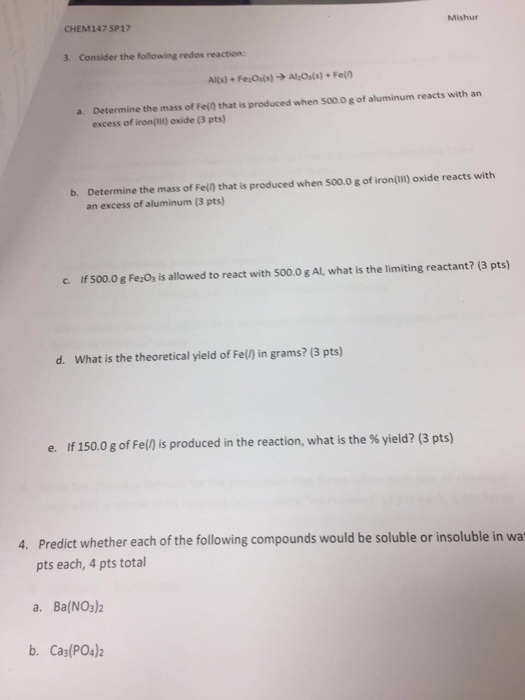Consider the following redox reaction: Al(s) + Fe_2O_3 rightarrow Al_2O_3(s) + Fe(l) a. Determine the mass of Fe(l) that is produced when 500.0 g of aluminum reacts with an excess of iron (III) oxide b. Determine the mass of Fe(l) that is produced when 500.0 g of iron (III) oxide reacts with an excess of aluminum c. If 500.0 g Fe_2O_3 is allowed to react with 500.0 g Al, what is the limiting reactant? d. What is the theoretical yield...

• ### a For the following balanced chemical equation, calculate how many moles of products would be produced...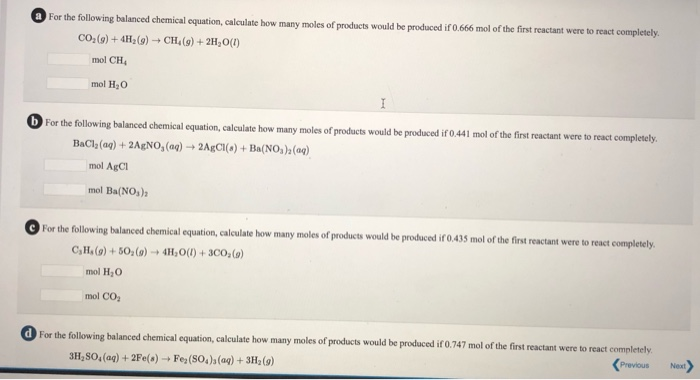a For the following balanced chemical equation, calculate how many moles of products would be produced if 0.666 mol of the first reactant were to react completely CO (9) + 4H2(g) + CH (9) + 2H, 0(1) mol CH mol H2O b For the following balanced chemical equation, calculate how many moles of products would be produced if 0.441 mol of the first reactant were to react completely. BaCl, (aq) + 2AgNO, (aq) + 2ACI(8) + Ba(NO), (aq) mol AgCI...

• ### How many moles of NaCI are produced when 0.5 moles of Na2COs react with excess hydrochloric...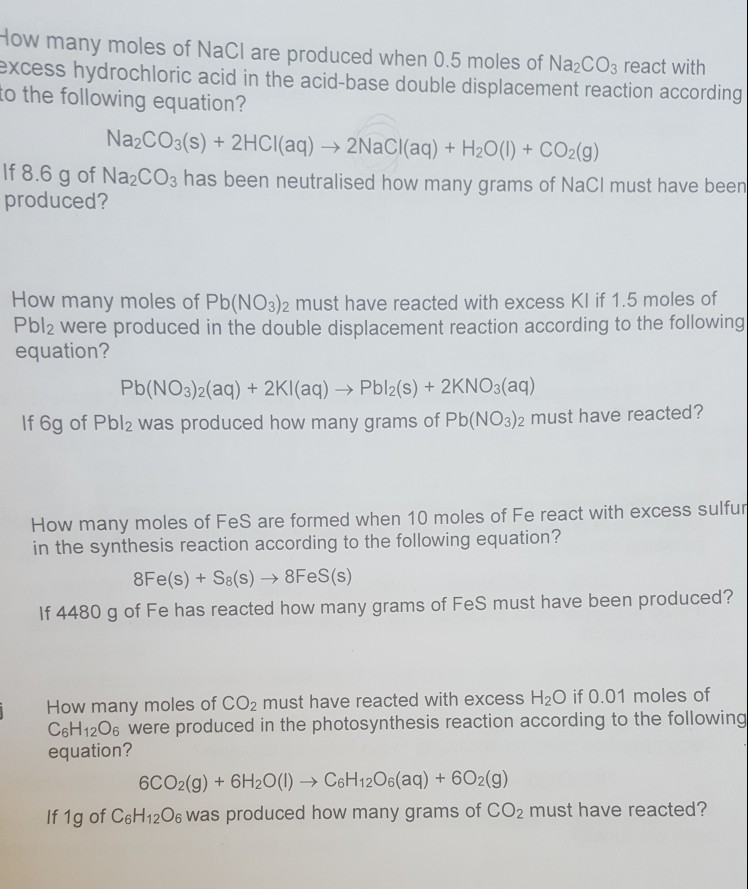How many moles of NaCI are produced when 0.5 moles of Na2COs react with excess hydrochloric acid in the acid-base double displacement reaction according to the following equation? Na2COs(s)+2HCl(aq) 2NaCl(aq)+ H20()+ CO2(9) 8.6 g of Na2COs has been neutralised how many grams of NaCI must have been produced? How many moles of Pb(NO3)2 must have reacted with excess KI if 1.5 moles of Pbl2 were produced in the double displacement reaction according to the following equation? Pb(NO3)2(aq) + 2Kl(aq)- Pbl2(s)...

• ### 4. From the balanced chemical equation: N2 + 3H2 2 NH3 a) Determine the number moles of NHs produced from the complete reaction of 2.5 moles of H:? [Spts] b) Using the above chemical equation...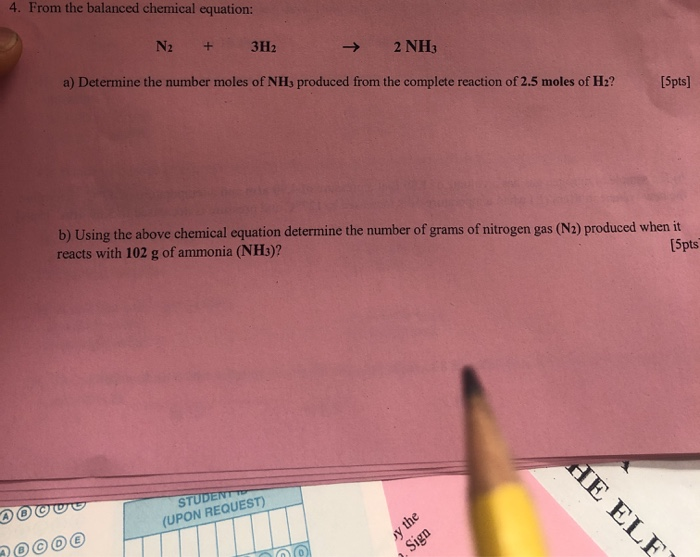4. From the balanced chemical equation: N2 + 3H2 2 NH3 a) Determine the number moles of NHs produced from the complete reaction of 2.5 moles of H:? [Spts] b) Using the above chemical equation determine the number of grams of nitrogen gas (N2) produced when it reacts with 102 g of ammonia (NH3)? [5pts (UPON REQUEST) 4. From the balanced chemical equation: N2 + 3H2 2 NH3 a) Determine the number moles of NHs produced from the complete reaction...

• ### Balance the chemical equation given below, and determine the number of grams of MgO needed to...

Balance the chemical equation given below, and determine the number of grams of MgO needed to produce 15.0 g of Fe2O3. ___ MgO(s) + ___ Fe(s) ? ___ Fe2O3(s) + ___ Mg(s)

• ### QUESTION 10 Balance the chemical equation given below, and determine the number of moles of iodine...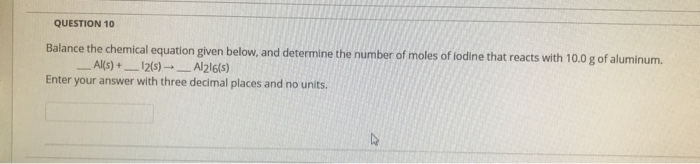QUESTION 10 Balance the chemical equation given below, and determine the number of moles of iodine that reacts with 10.0 g of aluminum. Al(s) + __-12(s) -_Al216(s) Enter your answer with three decimal places and no units.

• ### 2. a) Balance the equation below and use it to calculate the moles of Fe formed from 0.50 moles of Fe,O3: Fe2O3(...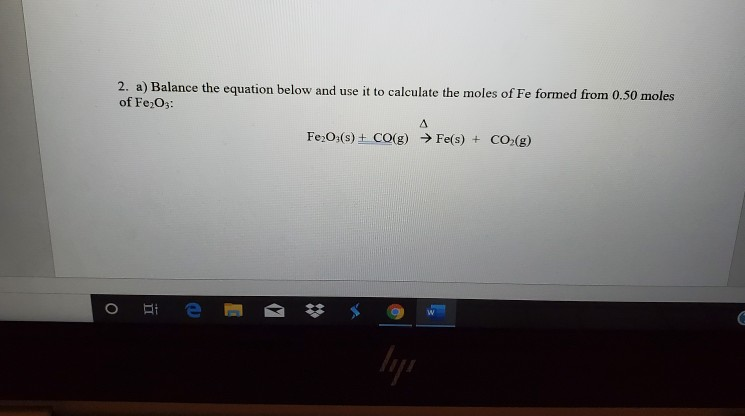2. a) Balance the equation below and use it to calculate the moles of Fe formed from 0.50 moles of Fe,O3: Fe2O3(s) + CO(g) → Fe(s) + CO2(g) 3. Balance the equation below then calculate the mass of ZnCl2 by obtained by reacting 10.0 g of zinc metal with an excess (more than is required) of hydrochloric acid Zn(s) + HCl(aq) → ZnCl2(aq) + H2(g) Morse backs pause

• ### Consider the following unbalanced chemical equation H2S(g)+O2(g)=SO2(g)+H2O(g) Determine the maximum number of moles of SO2 produced...

Consider the following unbalanced chemical equation H2S(g)+O2(g)=SO2(g)+H2O(g) Determine the maximum number of moles of SO2 produced from 10.00 moles H2S and 5.00moles of O2

• ### Part A How many moles of methane are produced when 85.1 moles of carbon dioxide gas...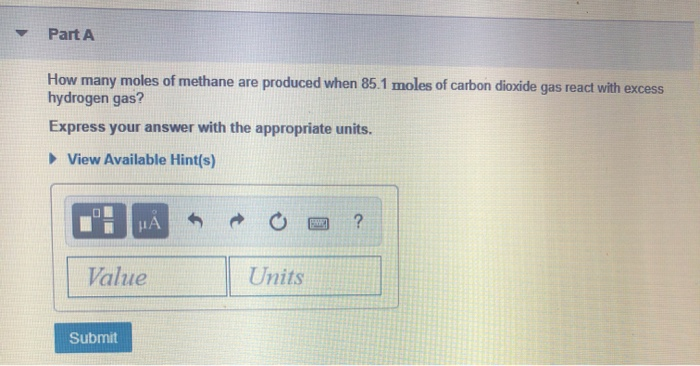Part A How many moles of methane are produced when 85.1 moles of carbon dioxide gas react with excess hydrogen gas? Express your answer with the appropriate units. View Available Hint(s) Value Units Submit Review Constants Periodic Table How many moles of hydrogen gas would be needed to react with excess carbon dioxide to produce 53.6 moles of water vapor? Express your answer with the appropriate units. View Available Hint(s) Value Units Submit

Free Homework App

Scan Your Homework
to Get Instant Free Answers
Need Online Homework Help?

Get Answers For Free
Most questions answered within 3 hours.